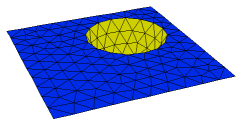﻿ Sample Problems > Usage > 3D_domains > regional_surfaces

# regional_surfaces

Navigation:  Sample Problems > Usage > 3D_domains >

# regional_surfaces{ REGIONAL_SURFACES.PDE

This problem demonstrates the use of regional definition of   3D extrusion surfaces.

There are three "REGIONS" defined, the cubical body of the domain, and two circular

patches. The circular patches each exist only on a single surface, and in no volumes.

The patch regions are used to define alternate extrusion surface shapes, and

insert two parabolic depressions in the top and bottom faces of the cube.

 Click "Domain Review" to watch the gridding process. }   title 'Regional surface definition'   coordinates    cartesian3   variables    Tp   definitions    long = 1       { domain size }    wide = 1    z1 = -1         { bottom surface default shape }    z2 = 1         { top surface default shape }    xc = wide/3     {some locating coordinates }    yc = wide/3    rc = wide/2    h = 0.8      K  = 1         { heat equation parameters }    Q = exp(-(x^2+y^2+z^2))   initial values    Tp = 0.   equations    Tp:  div(k*grad(Tp)) + Q = 0   extrusion z = z1,z2   boundaries   surface 1 value(Tp)=0   surface 2 value(Tp)=0     { define full domain boundary in base plane }   Region 1                 start(-wide,-wide)         value(Tp) = 0         line to (wide,-wide)           to (wide,wide)           to (-wide,wide)           to close     Limited region 2     { redefine bottom surface shape in region 2 }{ note that this shape must meet the default shape at the edge of the region }

z1 = -1+h*(1-((x+xc)^2+(y+yc)^2)/rc^2) { a parabolic dent }

surface 1   { region exists only on surface 1 }

start(-xc,-yc-rc) arc(center=-xc,-yc) angle=360

Limited region 3

{ redefine top surface shape in region 3 }

{ note that this shape must meet the default shape at the edge of the region }

z2 = 1-h*(1-((x-xc)^2+(y-yc)^2)/rc^2)

surface 2   { region exists only on surface 2 }

start(xc,yc-rc) arc(center=xc,yc) angle=360

plots

grid(x,y,z)

contour(Tp) on x=y

end